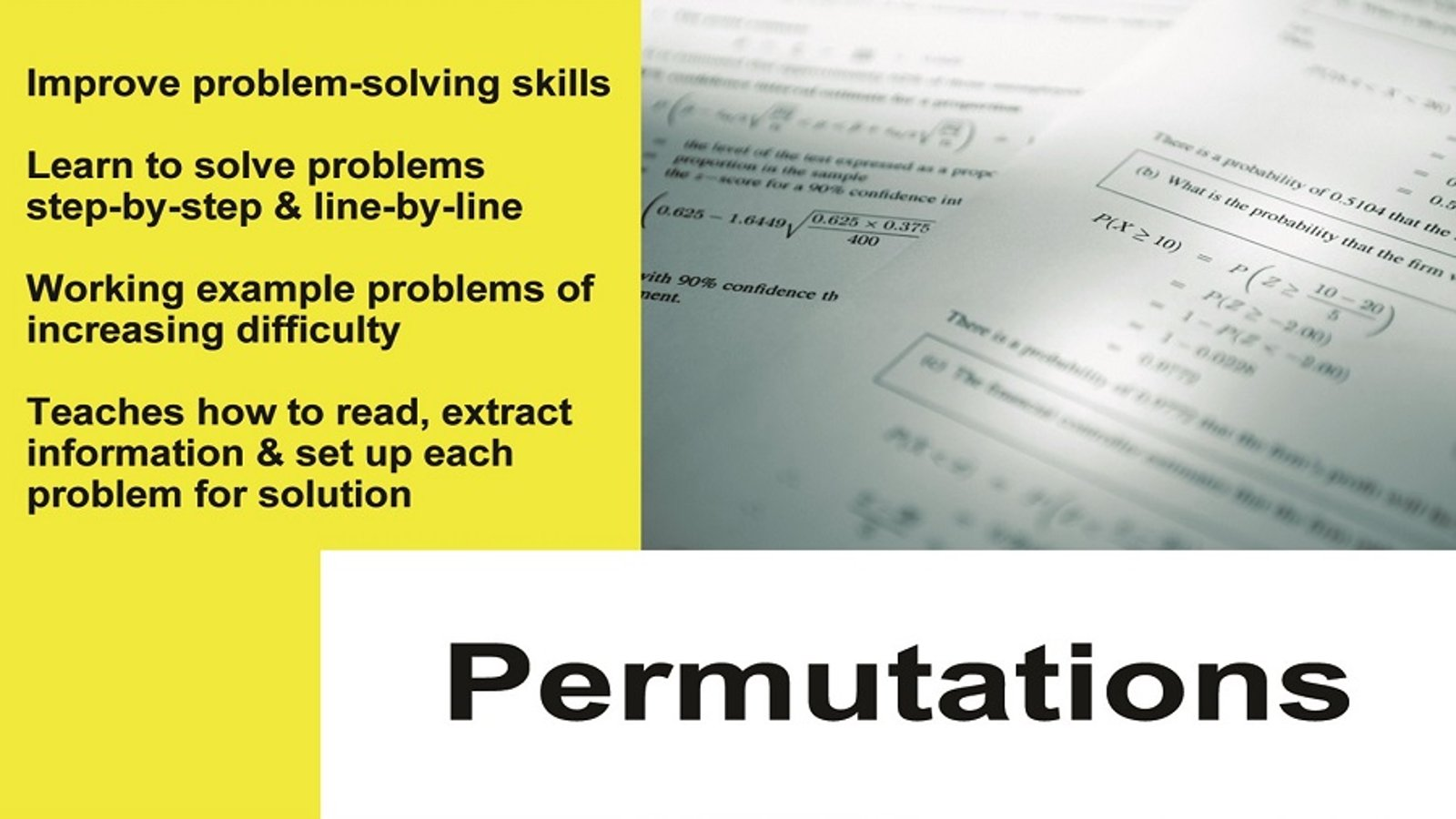Probability and Statistics Tutor Series

2011The Probability and Statistics Tutor is a complete 14 lesson series covering all of the core topics in detail. What sets this series apart from other teaching tools is that the concepts are taught entirely through step-by-step...

Supplier
TMW Media

In This Series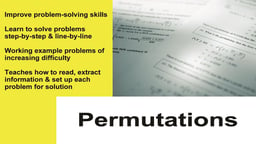Permutations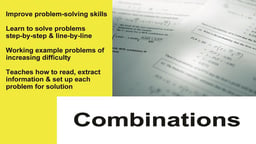Combinations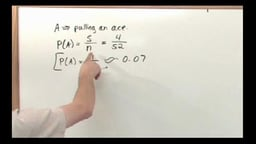Fundamentals of Probability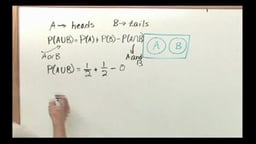Conditional Probability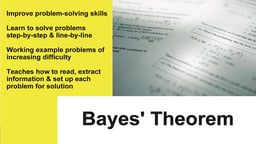Bayes' TheoremMathematical Expectation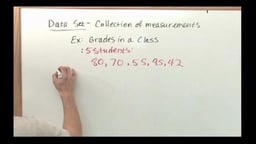Mean, Median and ModeStandard Deviation and Variance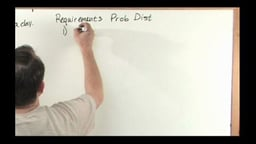Random Variables and Introduction to Probability Distributions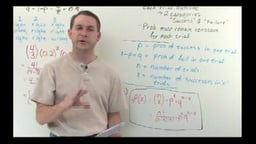Binomial Probability Distribution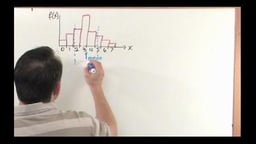Mean & Standard Deviation of the Binomial Distribution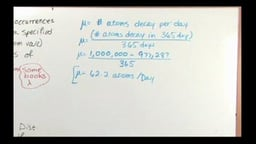Poisson Probability Distribution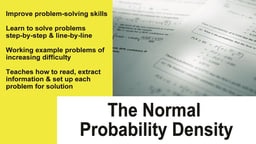Normal Probability Density